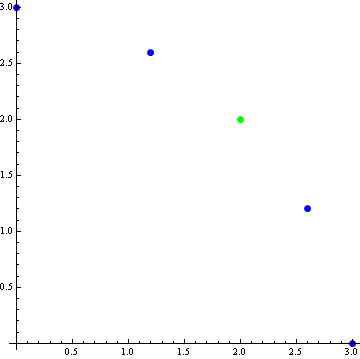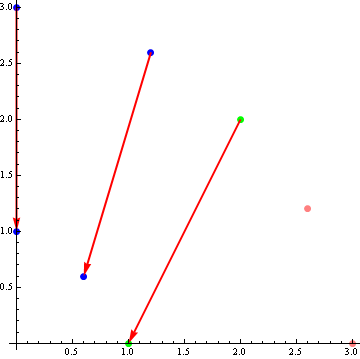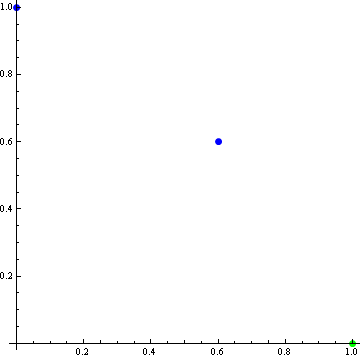# 0

Personal Blog

Back in the old days, when people were wise and the government was just, I did a post on the Nash bargaining solution for two player games. Here each player has their own utility function and they're choosing amongst joint options, and trying to bargain to find the best one. What was nice about this solution is that it is independent of irrelevant alternatives (IIA): once you've found the best solution, you can erase any other option, and it remains the best.

In order to do that, the Nash bargaining solution makes use of a "disagreement point", a special point that provides a zero to both utilities. This seems - and is - ugly. Can we preserve IIA without this clunky disagreement point?

By the title of the this post, you may have guessed that we can't. Specifically, assume the outcome is symmetric across both players (i.e. permuting the two utility functions preserves the outcome choice), the outcome is Pareto-optimal (any change will reduce the utility of at least one player) and there is no outside canonical choices for the utility functions (no special scales, no zeroes, no disagreement points). Then IIA must fail. It fails under weaker conditions as well, but the above lead to an easy picture-proof. And picture proofs are nice.

So assume there are five possible choices, whose utility values for the two players are (0, 3), (1.2, 2.6), (2, 2), (2.6, 1.2), (3, 0). These are graphed here:The choice set is symmetric and the green point (2, 2) is Pareto-optimal and on the axis of symmetry. Hence by the assumptions, the green point must be the outcome chosen. Now further assume IIA, and we will derive a contradiction.

First, by IIA, we can erase the losing points (2.6, 1.2) and (3, 0). Then we can rescale the utility functions: the utility function graphed on the x axis is divided by two, while the utility graphed on the y axis has 2 subtracted from it. These changes are illustrated here:This results in a final setup of (0, 1), (0.6, 0.6) and (1, 0):But this is obviously wrong: symmetry implies the correct outcome should be the blue point (0.6, 0.6), not the green (1, 0) which was the outcome before we removed the "irrelevant" extra points. We have derived a contradiction, and IIA must fall.

Personal Blog

New Comment